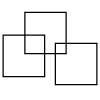#### You may also like### Three Squares

What is the greatest number of squares you can make by overlapping three squares?### Two Dice

Find all the numbers that can be made by adding the dots on two dice.### Biscuit Decorations

Andrew decorated 20 biscuits to take to a party. He lined them up and put icing on every second biscuit and different decorations on other biscuits. How many biscuits weren't decorated?

# Shut the Box

##### Age 5 to 7 Challenge Level:

There is no particular solution to this game.
Some people have tried other rules;
$1$. You can choose to turn over the numbers you've rolled, or add them together, or subtract one from the other. For example, if you were to roll a $5$ and $4$ you could choose to turn the $4$ and the $5$ over OR you could choose to add or subtract so that you could turn either the $9$ or the $1$ over.
$2$. When you came to a stop because you were unable to turn over new numbers, then those two dice numbers pass to the next player who can use them rather than rolling the dice again.
$3$. You can choose to turn over any set of cards that has the same total as the dice numbers you have thrown. For example, if you throw a 4 and 5, you could choose to turn over:
$4 & 5$ or $9$ or $1 & 8$ or $2 & 7$ or $3 & 6$ or $1 & 2 & 6$ or $1 & 3 & 5$ or $2 & 3 & 4$
$4$. You could multiply the two numbers on the dice together and then turn over any set of numbers which has that total. For example, if you throw 4 and 5, as well as the above, you could also choose to turn over:
$8 & 12$ or $9 & 11$ or $4 & 6 & 10$ or $1 & 2 & 3 & 4 & 10$, etc.
So, try these different versions of the game and different ways of scoring, and tell us what you think works best and why.